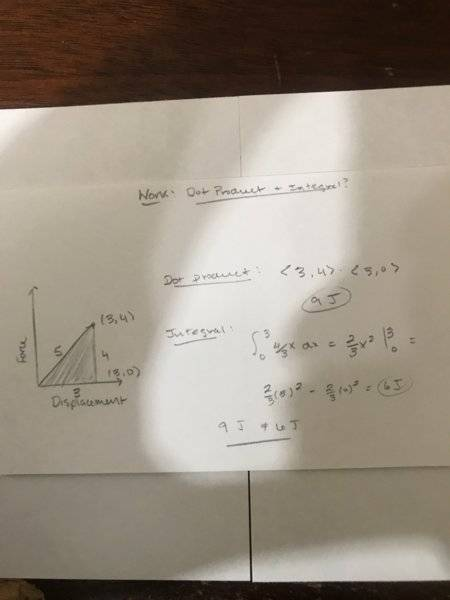# Work: Dot Product and Integral?

• learning_physica

#### learning_physica

I’m having trouble understanding the relationship between how work is both a dot product and integral. I know that work equals F • D and also the integral of F(x): the area under the curve of F and D.

However, let’s say that I have a force vector <3,4> and a displacement vector of <3,0>. The dot product yields 9J.

However, if I took the area under the curve by treating the vector <3,4> as a line segment which has a magnitude of 5 and the displacement vector also as a line segment with magnitude 3, then the area under the curve of this triangle with side lengths 3, 4, and 5 give me an area of 6J.

Does anyone know what I’m doing wrong?

You cannot use the hypotenuse as the displacement. If the force is ##\vec F = F_x~\hat x + F_y~\hat y +F_z~\hat z## and the displacement is ##\vec d =d_x~\hat x + d_y~\hat y +d_z~\hat z##, then the work done by this force is ##W=\vec F \cdot \vec d=F_xd_x+F_yd_y+F_zd_z##. There is no hypotenuse involved. When you have a force that depends on position, then the incremental work over a displacement ##d\vec s## is ##W=\vec F \cdot d\vec s=F_xdx+F_ydy+F_zdz## and you need to do 3 integrals to find the total work.

In the specific case that you posted, the work is just ##W=F_xx##. It turns out that ##F_x = F## only because the direction of the force has been chosen as the x-axis, but this does not mean that you can replace the displacement by its magnitude. You can do that only if both the force and the displacement are in the same direction.

The integral definition of work is a dot product. It is ##W = \int \vec F \cdot \vec{ds}##. If ##\vec F## is constant and the displacement is along a constant direction, then this becomes ##W = \vec F \cdot \int \vec {ds} = \vec F \cdot \vec D##

I’m having trouble understanding the relationship between how work is both a dot product and integral. I know that work equals F • D and also the integral of F(x): the area under the curve of F and D.
The integral is of the component of F along the path, which in this case is 3 N. So you're integrating a constant of 3 along ##ds## for 3 meters, which gives you 9 J.

However, let’s say that I have a force vector <3,4> and a displacement vector of <3,0>. The dot product yields 9J. However, if I took the area under the curve by treating the vector <3,4> as a line segment which has a magnitude of 5 and the displacement vector also as a line segment with magnitude 3, then the area under the curve of this triangle with side lengths 3, 4, and 5 give me an area of 6J.

Look, let's do it algebraically in terms of components.
$$W = \int \vec F \cdot \vec {ds} = \int \left( F_x i + F_y j \right) \cdot (i dx + j dy) = \int \left ( F_x dx + F_y dy \right ) \\ =\int F_x dx + \int F_y dy$$

F_x is a constant. Its plot vs x does not look like a triangle. F_y is a constant. Its plot vs y does not look like a triangle. You're confusing (x,y) space in some way with your plot of force vs distance.

For your example the first integral, the area under the curve for the x component, reduces to 3 * 3 = 9 J, and your second integral, the area under the curve for y, reduces to 0 * 4 = 0. Total 9 + 0 = 9 J.

•Dale
Could someone then tell me what’s wrong with my diagram#### Attachments

Could someone then tell me what’s wrong with my diagram
Your diagram is wrong in that it shows that the force varies with distance when it actually does not. Furthermore, if you want the area under the curve to be the work done by the force, you should plot Fx vs. x, not F vs. D.

Then, what would be an appropriate diagram @kuruman

Then, what would be an appropriate diagram @kuruman
As I indicated in post #6, plot Fx vs. x. Find Fx at different values of x, put the appropriate points on the graph and connect the dots.